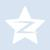## 在codecademy自学，有道题不会

anythinker 发布于 2017/03/15 17:45

Instructions

1. Define a function called `get_class_average`that has one argument `students`. You can expect `students` to be a list containing your three students.
2. First, make an empty list called `results`.
3. For each `student` item in the `class` list, calculate `get_average(student)` and then call `results.append()` with that result.
4. Finally, return the result of calling `average()`with `results`.

code:

tyler = {
"name": "Tyler",
"homework": [0.0, 87.0, 75.0, 22.0],
"quizzes": [0.0, 75.0, 78.0],
"tests": [100.0, 100.0]
}

# Add your function below!
def average(numbers):
return float(sum(numbers)) / len(numbers)

def get_average(student):
homework = average(student["homework"])
quizzes = average(student["quizzes"])
tests = average(student["tests"])
return 0.1 * homework + 0.3 * quizzes + 0.6 * tests

def get_letter_grade(score):
if (score >= 90):
return "A"
elif (score >= 80):
return "B"
elif (score >= 70):
return "C"
elif (score >= 60):
return "D"
else :
return "F"
def get_class_average(students):

0`results``=``[]`

`for` `s ``in` `students:`

`    ``results.append(get_average(s))`

`return` `average(results)`